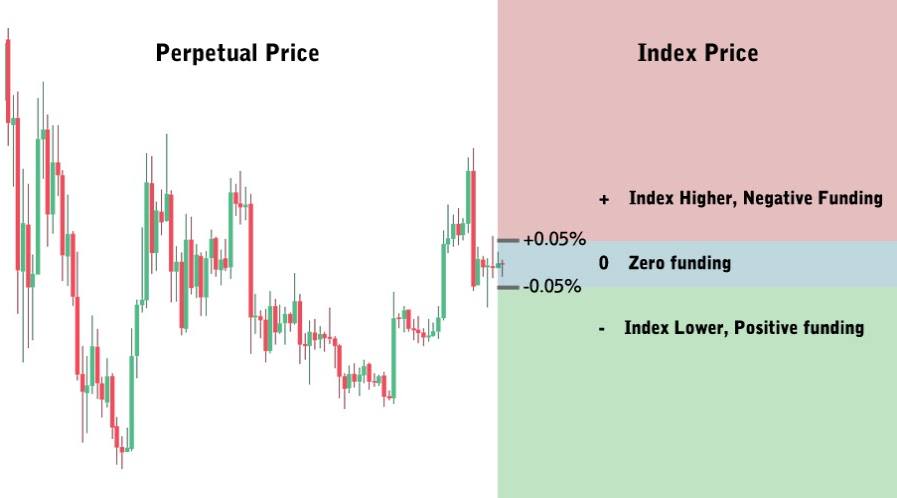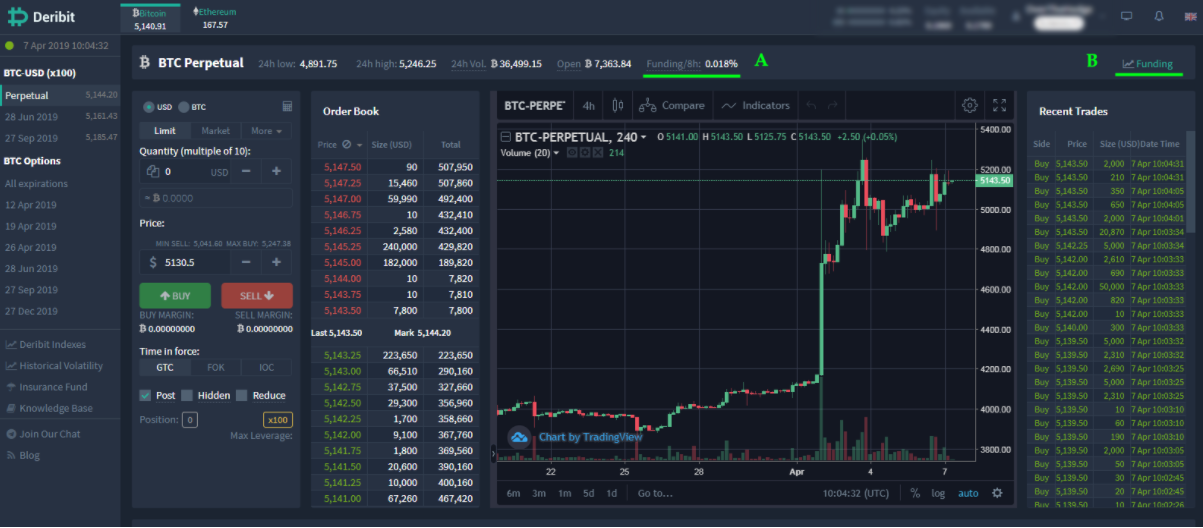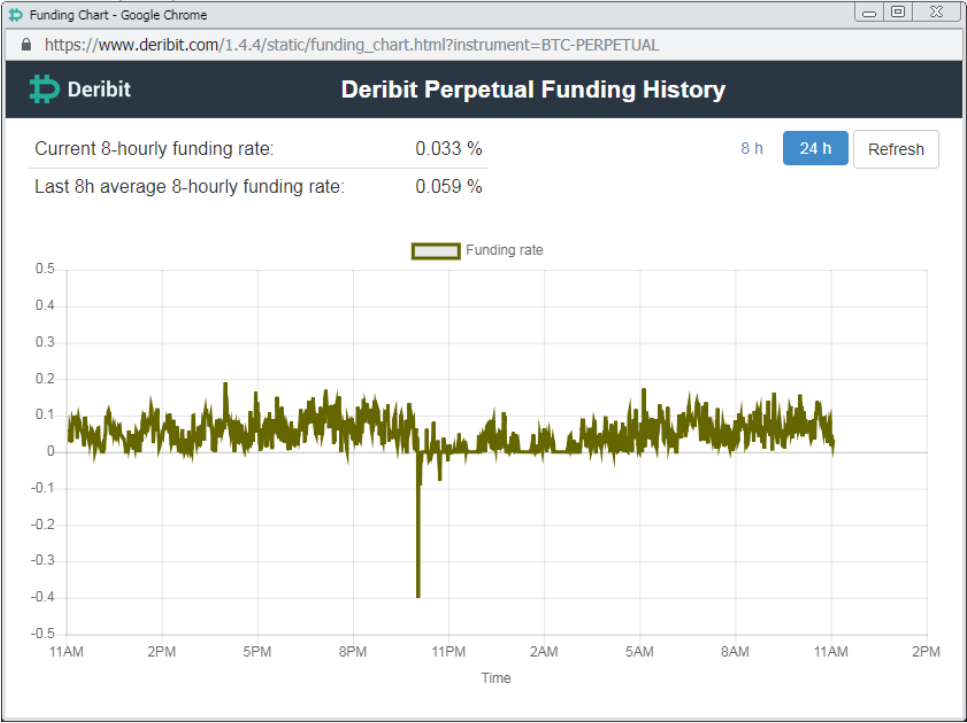Financial derivatives are designed to track the price of an underlying asset, for example Bitcoin or Ethereum in the crypto derivatives market.

Most traders are familiar with vanilla futures contracts. These contracts have a fixed expiry date, at which time the trader is obligated to sell or buy the underlying asset. Due to this fixed expiration date, the price of the futures contracts tends to track the price of the underlying asset. Futures contracts can of course trade at a discount or a premium to the underlying asset, however due to the fact that at expiration they will be settled using the underlying spot price, any difference will converge to zero as the time to expiration decreases.

Perpetual swaps do not have an expiry date, therefore they need another mechanism to keep the price of the contract as close to the index price as possible. This mechanism is called – the funding rate.

## How funding works

Funding is a payment made from one side of the trade (long or short) to the other. When the funding rate is negative, shorts pay longs, when funding is positive longs pay shorts.

When the price of the perpetual swap is lower than the index, then in order to strengthen demand for longs and subsequently encourage the price to increase towards the index, longs are paid funding by shorts (negative funding). This has the effect of decreasing demand for shorts and increasing demand for longs until the price approaches the index again.

Conversely, when the price of the perpetual swap is higher than the index, then in order to strengthen demand for shorts and subsequently encourage the price to fall towards the index, shorts are paid funding by longs (positive funding). This has the effect of decreasing demand for longs and increasing demand for shorts until the price reaches the price of the index.The further away from the index the price of the perpetual gets, the larger the funding rate becomes. It can increase up to a maximum of +/- 0.5%, as expressed per 8 hour interest rate. As this mechanism is only needed when the price is diverging too far from the index, if the perpetual price is within +/- 0.05% of the index, the funding rate is set to zero.

## Where to find the funding rate on Deribit?

When you are on the screen for the Perpetual swap the current funding rate is displayed at point A as shown below:You can also click ‘Funding’ at point B to open the funding rate pop up chart:On this screen you can see the current funding rate, the average rate over the last 8 hours and a chart displaying how the funding rate has changed over the last 8 or 24 hours.

You will notice how all the rates quoted are 8 hour rates. The 8 hour rate is displayed to make comparison simpler, and is just the amount you would pay/receive if the funding rate remained the same for 8 hours. However it’s actually calculated in real time and transferred every few seconds, therefore in reality it is a small time based fraction of the 8 hour rate.

## No fees on funding

The funding mechanism has one purpose and that is to keep the price in line with the index. Deribit does not charge any fees on funding and all funding is transferred directly between the parties trading the perpetual contracts. This makes the funding a zero sum game, where longs receive all funding from shorts, or shorts receive all funding from longs.

## Funding Rate

Sequentially, the funding rate is derived from the premium rate by applying a damper.

If the premium rate is within -0.05% and 0.05% range, the actual funding rate will be reduced to 0.00%.

If the premium rate is lower than -0.05%, then the actual funding rate will be the premium rate + 0.05%.

If the premium rate is higher than 0.05%, then the actual funding rate will be the premium rate – 0.05%.

Additionally, the funding rate is capped at +/- 0.5%, expressed as an 8-hour interest rate for BTC, and+/- 1% for ETH.

Funding Rate = Maximum (0.05%, Premium Rate) + Minimum (-0.05%, Premium Rate)

## The Full Funding Rate Calculation

Calculations of the funding rate for the Bitcoin Perpetual and Ethereum Perpetual are identical.

The funding rate percentage, expressed as an 8-hourly interest rate, is calculated as follows at any given point in time:

First, we calculate the Premium Rate:

Premium Rate = ((Mark Price – Deribit Index) / Deribit Index) * 100%

Second, we calculate the Funding Rate:

From the Premium Rate, the Funding Rate can be calculated by applying a dampener. If the Premium Rate is within -0.05% and 0.05%, the actual Funding Rate will be reduced to zero.

If the premium rate is lower than -0.05%, then the actual funding rate will be the Premium rate + 0.05%.

If the premium rate is higher than 0.05%, then the actual funding rate will be the Premium rate – 0.05%.

Additionally, the funding rate is capped at +/- 0.5%, expressed as an 8-hour interest rate for BTC, and+/- 1% for ETH.

Funding Rate = Maximum (0.05%, Premium Rate) + Minimum (-0.05%, Premium Rate)

Finally we calculate the Time Fraction:

Time Fraction = Funding Rate Time Period / 8 hours

The actual Funding Payment is calculated by multiplying the Funding Rate by the position size in BTC and the Time Fraction.

Funding Payment = Funding Rate * Position Size BTC * Time Fraction

For the full funding rate calculations and more examples you can view the Deribit knowledge base here.

AUTHOR(S)Cryptarbitrage